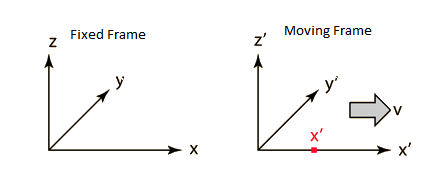# Lorentz Factor FormulaThe diagram shows the fixed frame in 3-dimensional view, with 3 directions x, y and z respectively.

Consider the Moving frame where x’ is moving with the primed frame with the velocity v in the x

direction.

The reference frames coincide at t=t’=0.

$$\begin{array}{l}{x}’=\frac{x-vt}{\sqrt{1-\frac{v^{2}}{c^{2}}}}\end{array}$$

$$\begin{array}{l}{y}’=y\end{array}$$

$$\begin{array}{l}{z}’=z\end{array}$$

$$\begin{array}{l}{t}’=\frac{t-\frac{vx}{c^{2}}}{\sqrt{1-\frac{v^{2}}{c^{2}}}}\end{array}$$

Where,

$$\begin{array}{l}\beta =\frac{v}{c}\end{array}$$

$$\begin{array}{l}\gamma =\,Lorentz\,factor\end{array}$$

$$\begin{array}{l}\gamma =\frac{1}{{{\sqrt{1-\frac{v^{2}}{c^{2}}}}}}\end{array}$$

For more such scientific information stay tuned with BYJU’S!!